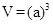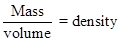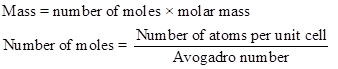Chapter 12.2, Problem 12.3CYU

Chapter
Section
Textbook Problem

Potassium chloride has the same unit cell as NaCl. Using the ion sizes in Figure 7.11, calculate the density of KCl.FIGURE 7.11 Relative sizes of some common ions. Rodii are given in picometers (1 pm 1 × 10‒12 m). (Data taken from J. Emsley, The Elements, Clarendon Press, Oxford, 1998, 3rd edition.)

Interpretation Introduction

Interpretation:

The density of potassium chloride has to be calculated.

Concept introduction:

The volume of the atom is given below,The density of the unit cell is calculated as follows,Explanation

One unit cell contains four potassium atom and four chlorine atom.

Still sussing out bartleby?

Check out a sample textbook solution.

See a sample solution

The Solution to Your Study Problems

Bartleby provides explanations to thousands of textbook problems written by our experts, many with advanced degrees!

Get Started

Find more solutions based on key concepts